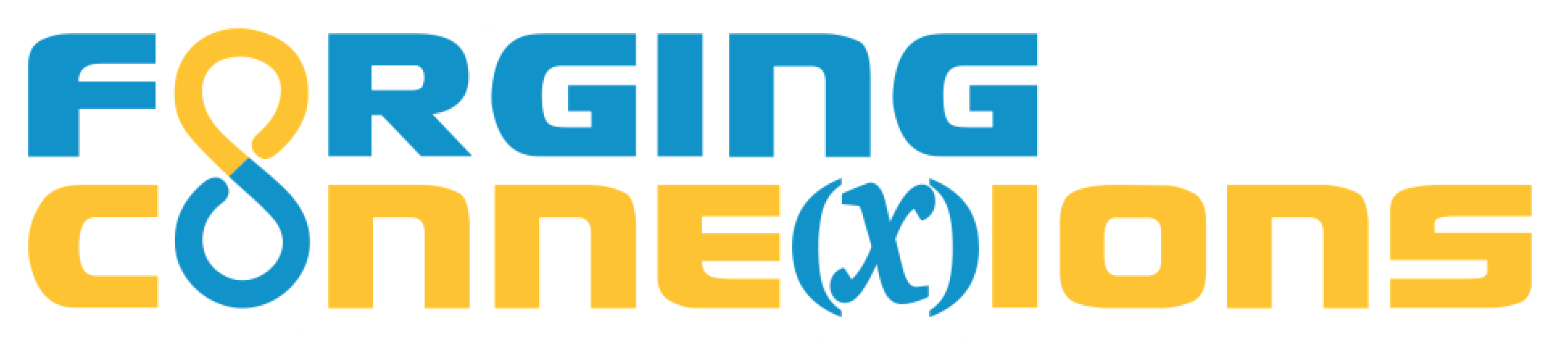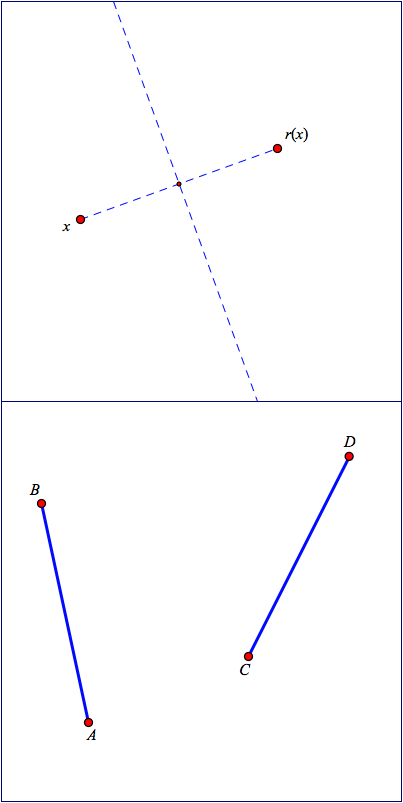Reflection Fixed Points

1 Warm-Up

On page 1, write down what you notice and wonder. (Don’t use the Distance tool yet.)

On page 2, measure some distances. Then write down what you notice and wonder about the distances.

With only the two distances shown above, how can you drag x to make it equally distant from y and r(y)?

What did you notice and wonder, when you tried to do this?

2 Find the Fixed Points

Below, you can’t drag x. Construct a second reflection. (It will use the same mirror.) Then use tracing to find the fixed points.

Below, use the first two tools to construct the line of all the fixed points. Use the Compass tool to check your answer.

3 Construct The Mirror

3a The Two-Circle Construction

As you already know, a reflect function’s fixed-point line is the same as its mirror line.

On the left, use the Compass twice and the Line tool once to construct the line of all the fixed points. Use the Compass tool again to check your answer.

On the right, use the same method to construct the perpendicular bisector of \overline{AB}. Drag A or B to check your construction. Then try the experiment suggested on page 2.

Hint video

Now that you’ve done the two-circle construction, can you explain why it works? How do you know for sure that the two points you used to construct the line are both fixed points of the reflect function?

3b A Line with Four Identities

• It’s the mirror line that defines the reflect function that takes x to r(x).
• It’s the locus of all the fixed points of the function that takes x to r(x).
• It’s the perpendicular bisector of the segment between x and r(x).
• It’s the line constructed using the intersections of the two circles defined by points x and r(x).

Use the tools to construct and/or trace all of these on the same page. The tools include Hide/Show buttons you can use to hide or show each way of constructing or tracing this line.

In your own words, describe something that's interesting or important about each of these four lines—four lines that are all the same, but also different, each in its own way!

X

4 Patty Paper ReflectionClick the image on the left to print it full size, or get a copy from your teacher. The top printed diagram shows x, r(x), and the constructed line of fixed points. Trace points x and r(x) onto a piece of patty paper. Fold the patty paper so that x lies on top of r(x).

✏️ How does the patty-paper fold line relate to the fixed points of the reflect function connecting x and r(x)?

Use the bottom printed diagram to trace \overline{AB} and \overline{CD} on a new sheet of patty paper. Fold the patty paper to reflect A onto C, trace the reflected location of \overline{AB}, and label the reflected endpoints A' and B'.

Unfold your paper. Then make a new fold to put B' on top of D.

✏️ What do you notice? What do you wonder?

5 The Perpendicular Bisector Theorem

Investigate Distances

In this sketch, construct a segment and its perpendicular bisector.

Label the segment \overline{AB}, and label the perpendicular bisector m.

Construct a point P on the perpendicular bisector. Then construct \overline{PA} and \overline{PB}, and measure their lengths.

✏️ What do you notice? What do you wonder?

✏️ Is your observation always true? To check, drag P to different locations on m, and drag A and B around to change the location and length of \overline{AB}.

✏️ Write your conclusion down as an “If a point ... then the distance(s) ...” statement. This is the perpendicular bisector theorem.

Prove the Theorem

It’s one thing to make a statement about distances in a geometric figure. It’s quite another thing to be able explain why that statement must be true, to demonstrate the logic behind it, to truly understand the mathematics that makes it true.

In this sketch you’ll work out the steps to prove the theorem.

Begin by constructing the same point P and the same segments \overline{PA} and \overline{PB} as you did above.

How can you use the remaining tools to illustrate the perpendicular bisector theorem?

What is the effect of each tool?

How do these tools work together to show that the perpendicular bisector theorem must be true?

6 The Converse of the Perpendicular Bisector Theorem

The perpendicular bisector theorem says, "If point P is on the perpendicular bisector of \overline{AB}, then the lengths of \overline{PA} and \overline{PA} are equal.

What happens if you turn this statement around? If the lengths of \overline{PA} and \overline{PA} are equal, what does that tell you about P, A, and B?

If a point is equally distant from two other points, it lies on the perpendicular bisector of the segment connecting those two points.

Coming soon...

## Moved temporarily from Reflect Family; still need to be integrated here.

7 Trace the Fixed Points

Independent variable x and its reflected image r_j(x) are shown, but the function rule is hidden.

Your job is to find it.

• Drag independent variable x to find a fixed point of the function.
• Continue dragging x to find more fixed points.
• Turn on tracing for both points and drag again to trace the whole mirror.

✏️ How do you know that the fixed points you traced are actually points of the mirror?

8 Fixed Points and Distances

Below left, measure the distance from w to each of the other points, and drag w to make the two distances nearly equal. Turn tracing on and drag w to show all the places where the distances are nearly equal.

Below right, construct a circle with its center at w and its radius point at x. Then drag w so the circle touches r(x). Turn tracing on and drag w to show all the places where the circle touches both x and r(x).

✏️ In each sketch, what do the traces have to do with the fixed points of the reflect function r? Explain the connection.

9 Reflect Shapes

With this sketch, you can reflect a variety of shapes.

1. Construct one of the shapes.
2. Using the Reflect tool, attach x to your shape.
3. Use the Animate tool to animate your independent variablex along the shape

Note that the Reflect tool in this sketch constructs three things: the independent variable x, the mirror, and the dependent variable r_j(x).

• Page 1: Experiment with reflecting shapes.
• Page 2: Reflect to m,atch the given letter shape.
• Page 3: Reflect to create other letter shapes.
• Page 4: Experiment on your own.

10 Find the Mirror

This sketch challenges you to find the hidden mirror(s). On pages 1, 2, and 3, your job is to find the mirror so that if you animate x on the domain, r_j(x) will move around the range. Pages 4 and 5 provide slightly different challenges.

✏️ What did you notice, and what did you wonder, as you constructed these reflect functions?.

## End of Moved from Reflect Family materials...

X

11 Objectives

This page supports the Reflection Fixed Points lesson from the Introducing Geometric Transformations as Functions unit. After investigating and experimenting with the fixed points of the reflect function, if you are given two points you will be able to construct the mirror that relates them, and if you are given a mirror and a single point you will be able to construct the other point. You will learn some important facts about distances between points, and you will be able to describe and explain the perpendicular bisector theorem and its converse.

By the end of this lesson, you’ll be able to perform these actions and answer these questions:

• To be written.

The Fine Print

Requirements:

These activities require web access using a browser that supports HTML5 and JavaScript. (That means almost any current browser.) No purchase is required, and there’s no advertising anywhere.

Release Information

Update History:

Record every major revision, in reverse chronological order

21 Mar 2019: First draft of this lesson.